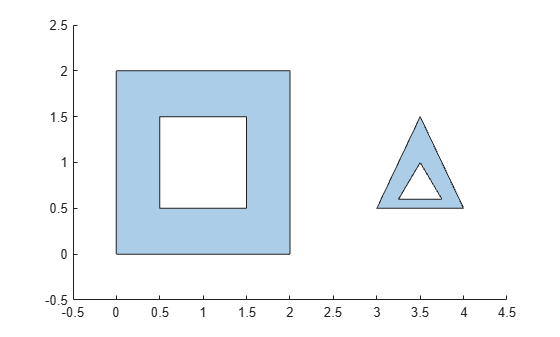holes

Convert polyshape hole boundaries to array of polyshape objects

Description

example

polyout = holes(polyin) returns the hole boundaries of a polyshape object as an array of polyshape objects. The number of elements in polyout is equal to the number of hole boundaries in polyin.

Examples

collapse all

Create a polygon containing two solid regions and two holes. Convert the hole boundaries to an array of polyshape objects.

P = [0 0; 0 2; 2 2; 2 0; NaN NaN; 0.5 0.5; 0.5 1.5; 1.5 1.5; 1.5 0.5; ...
NaN NaN; 3 0.5; 3.5 1.5; 4 0.5; NaN NaN; 3.25 0.6; 3.75 0.6; 3.5 1];
polyin = polyshape(P);
plot(polyin)
xlim([-0.5 4.5])
ylim([-0.5 2.5])polyout = holes(polyin)
polyout =
2x1 polyshape array with properties:

Vertices
NumRegions
NumHoles

plot(polyout)
xlim([-0.5 4.5])
ylim([-0.5 2.5])Input Arguments

collapse all

Input polyshape, specified as a scalar.

Data Types: polyshape